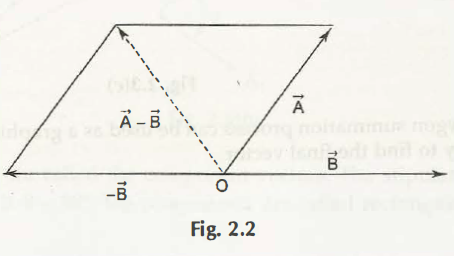Vector allows understanding absolute value of magnitude and direction. The vector helps to identify any mathematical object which is defined as any directed line segment. Few examples of vector quantities are velocity acceleration, angular momentum, displacement and velocity acceleration.

Vector is geometrically represented by arrow. Length of any arrow is proportional to that of magnitude of vector. Through head of an arrow it helps to get the sense of direction.

Displacement vector is represented as arrow. The tail of vector is A and the head is B. the length is further measured as AB which is a magnitude of vector.

Before performing any subtraction it is important to define negative of vector. Negative of any vector is the other vector which comes along with same magnitude but it is in opposite direction.Links of Previous Main Topic:-

Links of Next Mechanical Engineering Topics:-

### Submit Your Assignment### Customer Reviews

My Homework Help
Rated 5.0 out of 5 based on 510 customer reviews at